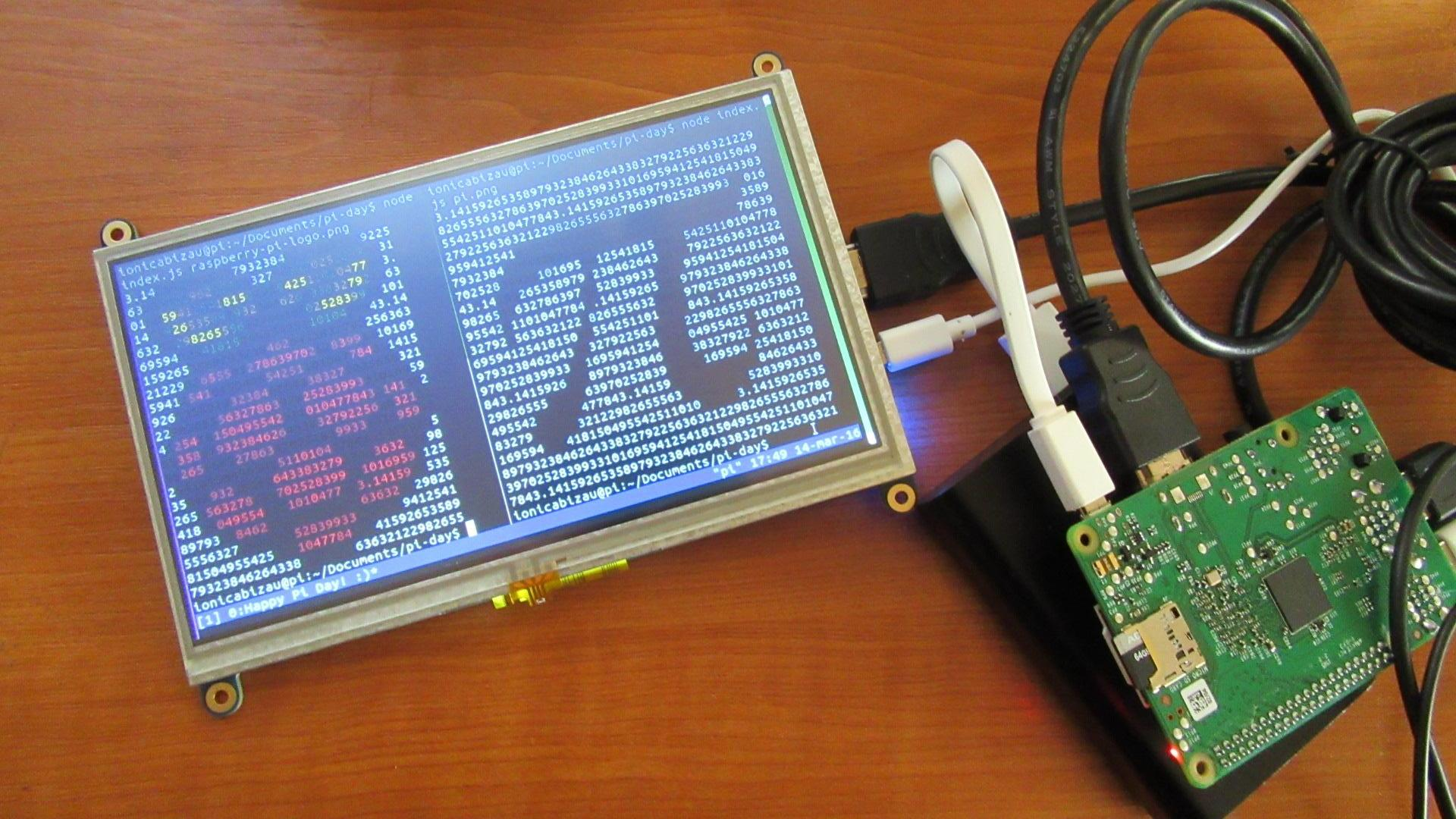# Pi Day, Raspberry Pi and Command Line

Everyone knows (or should know) what the Pi number is. Basically, its value is defined by the division of a circle circumference to its diameter. That's the Pi number! The nice thing about it is its irrational nature. That means it has a lot an infinity of decimals.

Usually, Pi is approximated as 3.14. And today is 14th of March! So, happy Pi day! :)

I have a Raspberry Pi computer around, and I was thinking to use two of my libraries to create something nice: displaying the Raspberry Pi's logo and the Pi number in the command line: it's funny how it basically stands for Raspberry Pi (the raspberry image and the pi number). I wanted one more thing: using the pi number decimals in the output characters. Here's the result (see below how I did it):## How I did it

To display the images in the terminal, I used `image-to-ascii`. To pass different image urls, I decided to use command line arguments.

To get the first n decimals of the pi number, I used another module I created this time last year: `pi`. This module returns a good approximation of pi:

``````const pi = require("pi");

console.log(pi(10));
// => '3.141592653'
``````

So, I created a file named `index.js` and I wrote the following stuff in it (follow the inline comments):

``````// Require the needed dependencies
// `pi` will be used to return the first `n` decimals of pi
const pi = require("pi")

// image-to-ascii for displaying the images in the terminal
, img = require("image-to-ascii")

// We use this module to stringify the pixel matrix after
// modifying the internal data (basically, the characters)
, stringify = require("asciify-pixel-matrix")
;

// Take the image url/path from the command line arguments
img(process.argv, {
// We turn off the stringifying, since we really want to do
// some changes before displaying the images
stringify: false
, concat: false
}, (err, converted) => {
// Handle the errors
if (err) { return console.error(err); }

// `converted` is an array of arrays (in fact, a matrix of pixels)
// We use the `converted` matrix to know how many decimals we
// need: width x height
// `piNumber` will be a string in this format: "3.14...." (with a
// lot of decimals)
var piNumber = pi(converted.length * converted.length);

// We will use this `i` variable to get the current index
var i = -1;

// For each row in the matrix
converted.forEach(cRow => {
// ...and for each pixel in the row
cRow.forEach(px => {
// ...update the character using a pi decimal, in order
px.char = piNumber[i = ++i % piNumber.length];
});
});

// Finally, stringify everything and display the result! Yay!
console.log(stringify.stringifyMatrix(converted));
});
``````

The requirements to run this script are:

• Node.js (I installed it on my Raspberry Pi using `nvm`)
• `graphicsmagick`: `sudo apt-get install graphicsmagick` (this is optional, kind of: if it's not available `image-to-ascii` will compile some C/C++ stuff, but it will probably take a long time)
• …and of course the `npm` dependencies: `npm i image-to-ascii pi asciify-pixel-matrix`

Happy Pi Day!

PS: I posted this article using my Raspberry Pi, connected to the Internet and using a 7" display. Just perfect. :)